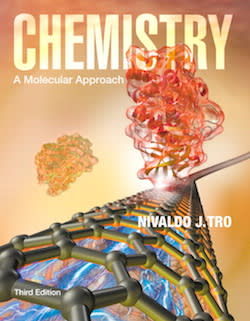Chemistry Chemistry: A Molecular ApproachChemistry: A Molecular Approach Tro • 3rd Edition • 978-0321809247

Not the textbook you were looking for? Pick another one here.

Ch.3 - Molecules, Compounds & Chemical Equations

Metal Alloys Polyatomic Ions Naming Covalent Compounds Naming Ionic Compounds Naming Acids Empirical Formula Combustion Analysis Mass Percent Formula Balancing Chemical Equations Functional Groups in Chemistry

BONUS: Mathematical Operations and Functions

Multiplication and Division Operations Addition and Subtraction Operations Power and Root Functions Logarithmic and Natural Logarithmic Functions The Quadratic Formula

BONUS: Lab Techniques and Procedures

Laboratory Materials Experimental Error Distillation & Floatation Chromatography Filtration and Evaporation Extraction Test for Ions and Gases

Ch.4 - Chemical Quantities & Aqueous Reactions

Stoichiometry Molarity Solution Stoichiometry Normality & Equivalent Weight Solubility Rules Net Ionic Equations Electrolytes Redox Reaction Activity Series End of Chapter 4 Problems

Ch.6 - Thermochemistry

Internal Energy Calorimetry Hess's Law Enthalpy of Formation End of Chapter 6 Problems

Ch.9 - Chemical Bonding I: The Lewis Model

Chemical Bonds Lattice Energy Born Haber Cycle Dipole Moment Lewis Dot Structure Octet Rule Formal Charge Resonance Structures

Ch.10 - Molecular Shapes & Valence Bond Theory

Molecular vs Electron Geometry Bond Angles Hybridization Molecular Orbital Theory Heteronuclear Diatomic Molecules

Ch.11 - Liquids, Solids & Intermolecular Forces

Molecular Polarity Intermolecular Forces Phase Diagram Heating and Cooling Curves Unit Cell Clausius-Clapeyron Equation

Ch.13 - Chemical Kinetics

Rate of Reaction Average Rate of Reaction Arrhenius Equation Rate Law Integrated Rate Law

Ch.14 - Chemical Equilibrium

Chemical Equilibrium ICE Chart Le Chatelier's Principle The Reaction Quotient

Ch. 17 - Chemical Thermodynamics

Spontaneous Reaction First Law of Thermodynamics Entropy Gibbs Free Energy

Ch.18 - Electrochemistry

Redox Reaction Balancing Redox Reaction The Nernst Equation Faraday's Constant Galvanic Cell Batteries and Electricity

Ch.19 - Nuclear Chemistry

Radioactive Decay Band of Stability Magic Numbers First Order Half Life

Ch.24 - Transition Metals and Coordination Compounds

Transition Metals Transition Metals Properties Coordination Complexes Naming Coordination Compounds Coordination Isomers